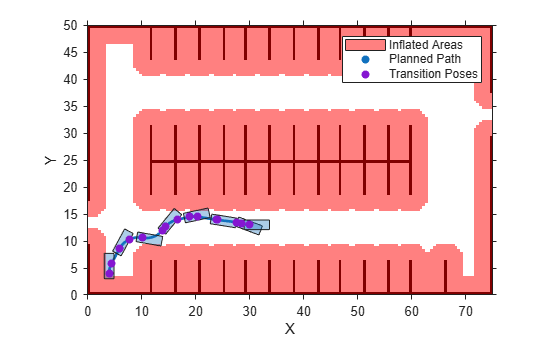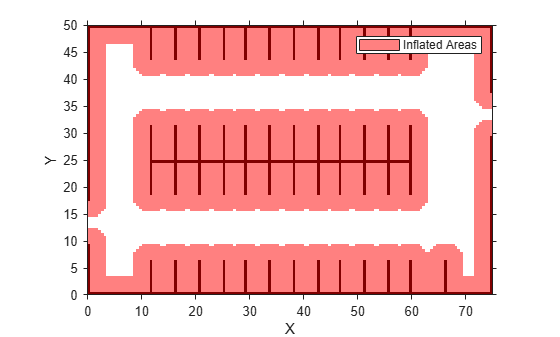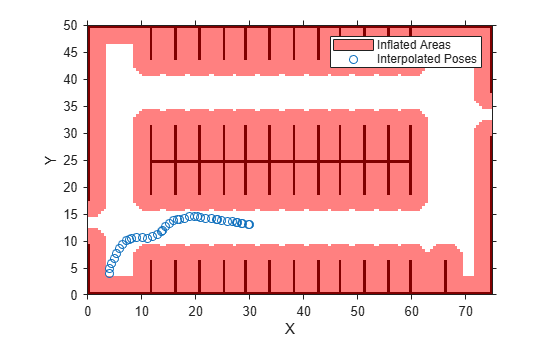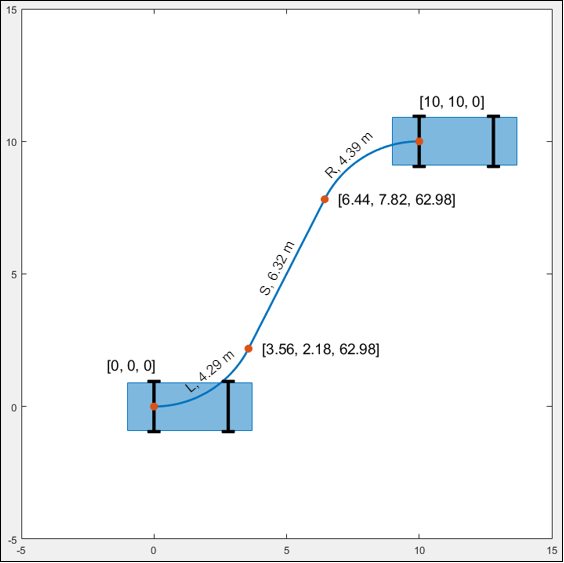interpolate

Interpolate poses along planned vehicle path

Description

example

poses = interpolate(refPath) interpolates along the length of a reference path, returning transition poses. For more information, see Transition Poses.

example

poses = interpolate(refPath,lengths) interpolates poses at specified points along the length of the path. In addition to including poses corresponding to specified lengths, poses also includes the transition poses.

[poses,directions] = interpolate(___) also returns the motion directions of the vehicle at each pose, using inputs from any of the preceding syntaxes.

Examples

collapse all

Plan a vehicle path through a parking lot by using the optimal rapidly exploring random tree (RRT*) algorithm. Check that the path is valid, and then plot the transition poses along the path.

Load a costmap of a parking lot. Plot the costmap to see the parking lot and inflated areas for the vehicle to avoid.

costmap = data.parkingLotCostmap;
plot(costmap)Define start and goal poses for the vehicle as [x, y, Θ] vectors. World units for the (x,y) locations are in meters. World units for the Θ orientation angles are in degrees.

startPose = [4, 4, 90]; % [meters, meters, degrees]
goalPose = [30, 13, 0];

Use a pathPlannerRRT object to plan a path from the start pose to the goal pose.

planner = pathPlannerRRT(costmap);
refPath = plan(planner,startPose,goalPose);

Check that the path is valid.

isPathValid = checkPathValidity(refPath,costmap)
isPathValid = logical
1

Interpolate the transition poses along the path.

transitionPoses = interpolate(refPath);

Plot the planned path and the transition poses on the costmap.

hold on
plot(refPath,'DisplayName','Planned Path')
scatter(transitionPoses(:,1),transitionPoses(:,2),[],'filled', ...
'DisplayName','Transition Poses')
hold offPlan a vehicle path through a parking lot by using the rapidly exploring random tree (RRT*) algorithm. Interpolate the poses of the vehicle at points along the path.

Load a costmap of a parking lot. Plot the costmap to see the parking lot and inflated areas for the vehicle to avoid.

costmap = data.parkingLotCostmap;
plot(costmap)Define start and goal poses for the vehicle as [x, y, Θ] vectors. World units for the (x,y) locations are in meters. World units for the Θ orientation angles are in degrees.

startPose = [4, 4, 90]; % [meters, meters, degrees]
goalPose = [30, 13, 0];

Use a pathPlannerRRT object to plan a path from the start pose to the goal pose.

planner = pathPlannerRRT(costmap);
refPath = plan(planner,startPose,goalPose);

Interpolate the vehicle poses every 1 meter along the entire path.

lengths = 0 : 1 : refPath.Length;
poses = interpolate(refPath,lengths);

Plot the interpolated poses on the costmap.

plot(costmap)
hold on
scatter(poses(:,1),poses(:,2),'DisplayName','Interpolated Poses')
hold offInput Arguments

collapse all

Planned vehicle path, specified as a driving.Path object.

Points along the length of the path, specified as a real-valued vector. Values must be in the range from 0 to the length of the path, as determined by the Length property of refPath. The interpolate function interpolates poses at these specified points. lengths is in world units, such as meters.

Example: poses = interpolate(refPath,0:0.1:refPath.Length) interpolates poses every 0.1 meter along the entire length of the path.

Output Arguments

collapse all

Vehicle poses along the path, returned as an m-by-3 matrix of [x, y, Θ] vectors. m is the number of returned poses.

x and y specify the location of the vehicle in world units, such as meters. Θ specifies the orientation angle of the vehicle in degrees.

poses always includes the transition poses, even if you interpolate only at specified points along the path. If you do not specify the lengths input argument, then poses includes only the transition poses.

Motion directions of vehicle poses, returned as an m-by-1 vector of 1s (forward motion) and –1s (reverse motion). m is the number of returned poses. Each element of directions corresponds to a row of poses.

collapse all

Transition Poses

A path is composed of multiple segments that are combinations of motions (for example, left turn, straight, and right turn). Transition poses are vehicle poses corresponding to the end of one motion and the beginning of another motion. They represent points along the path corresponding to a change in the direction or orientation of the vehicle. The interpolate function always returns transition poses, even if you interpolate only at specified points along the path.

The path length between transition poses is given by the MotionLengths property of the path segments. For example, consider the following path, which is a driving.Path object composed of a single Dubins path segment. This segment consists of three motions, as described by the MotionLengths and MotionTypes properties of the segment.The interpolate function interpolates the following transition poses in this order:

1. The initial pose of the vehicle, StartPose.

2. The pose after the vehicle turns left ("L") for 4.39 meters at its maximum steering angle.

3. The pose after the vehicle goes straight ("S") for 6.32 meters.

4. The pose after the vehicle turns right ("R") for 4.39 meters at its maximum steering angle. This pose is also the goal pose, because it is the last pose of the entire path.

The plot shows these transition poses, which are [x, y, Θ] vectors. x and y specify the location of the vehicle in world units, such as meters. Θ specifies the orientation angle of the vehicle in degrees.Extended Capabilities

Topics

Introduced in R2018b